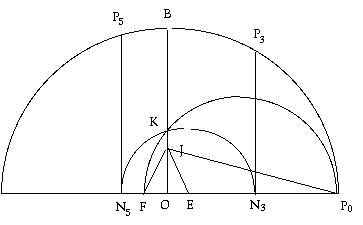Carl Friedrich Gauss was born April 30, 1777 and when he was 19 years old, he proved that there was a way to construct a 17 sided regular polygon with only a compass and straight edge–something that escaped the ancient Greek mathematicians. This is how it is done:1. Draw a circle around point O.

2. Draw a diameter to form a Semicircle P0

3. Find the perpendicular of OP0 and call it OB

4. Find J a quarter the way up OB (bisect OB a couple of times)

5. Join JP0 to form < OJP

6. Find E so that < OJE is a quarter of < OJP (Bisect is a couple of times)

7. Find F so the < EJF is 45° (bisect a perpendicular)

8. Construct a semicircle from F to P0 (bisect FP0 to find the center of the semicircle)

9. Find point K at the place this semicircle cuts across OB.

10. Draw semicircle with center E and radius EK

11. This cuts the extension of OP0 at N3 and N5

12. Draw the perpendiculars through N3 and N3 to find P3 and P5

13. P0, P3, P5 are the original, third and fifth points of the Heptagon.

Can you find a way to discover half the distance between P3 and P5 so you get the single length of one side? If you can then you can draw all 17 equal sides!